# 什么是注意力機制？# Encoder-Decoder 框架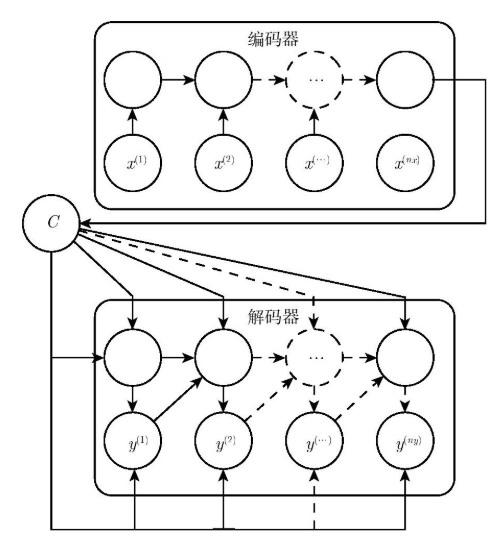$h_t = f(h_{t-1},x_t)\tag{1}$

$C=q(h_1,h_2,\cdots,h_{T_x})\tag{2}$

$C=q(h_1,h_2,\cdots,h_{T_x})=h_{T_x}\tag{3}$

$y_t=\arg \max P(y_t)=\prod_{t=1}^{T}p(y_t|y_1,y_2,\cdots,y_{t-1},C)\tag{4}$

$y_t=g(y_{t-1},s_{t-1},C)\tag{5}$

## 局限性

Encoder-Decoder 框架雖然應用廣泛，但是其存在的局限性也比較大。其最大的局限性就是 Encoder 和 Decoder 之間只通過一個固定長度的語義向量 $C$ 來唯一聯系。也就是說，Encoder 必須要將輸入的整個序列的信息都壓縮進一個固定長度的向量中，存在兩個弊端：一是語義向量 C 可能無法完全表示整個序列的信息；二是先輸入到網絡的內容攜帶的信息會被后輸入的信息覆蓋掉，輸入的序列越長，該現象就越嚴重。這兩個弊端使得 Decoder 在解碼時一開始就無法獲得輸入序列最夠多的信息，因此導致解碼的精確度不夠準確。

# Attention 機制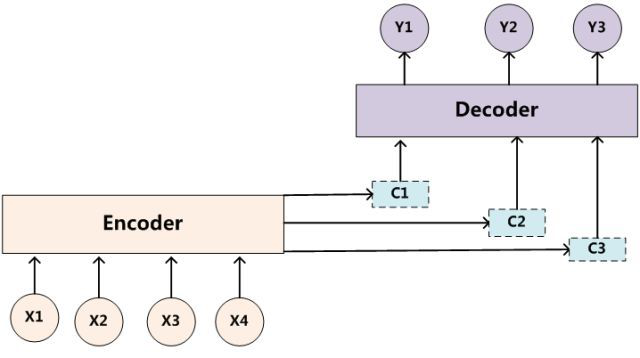$C_i=\sum_{j=0}^{T_x}{a_{ij}f(x_j)}\tag{6}$

$a_{ij}=\frac{exp(e_{ij})}{\sum_{k=1}^{T_x}exp(e_{ik})}\tag{7}$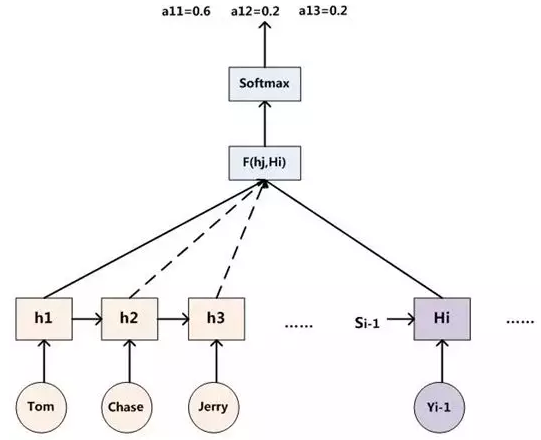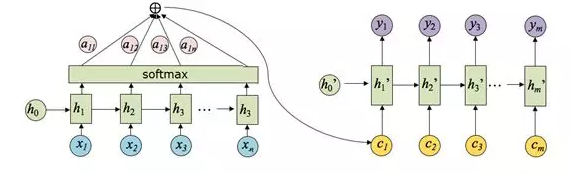# Attention 原理

Attention 機制的一個重點就是獲得 attention value，即機器翻譯中的語義編碼 $C_i$。在上一節中我們知道該值是通過輸入元素按照不同的權重參數組合而成的，所以我們可以將其定義為一個 attention 函數，比較主流的 attention 函數的機制是采用鍵值對查詢的方式，其工作實質如下圖所示：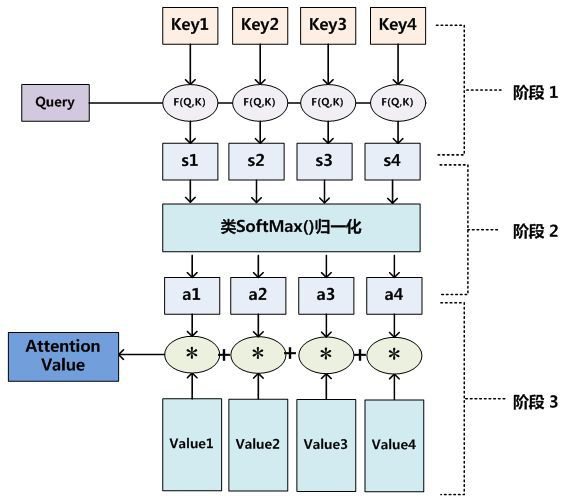Step 0：準備隱藏狀態Step 1：得到每一個 Encoder 隱藏狀態的得分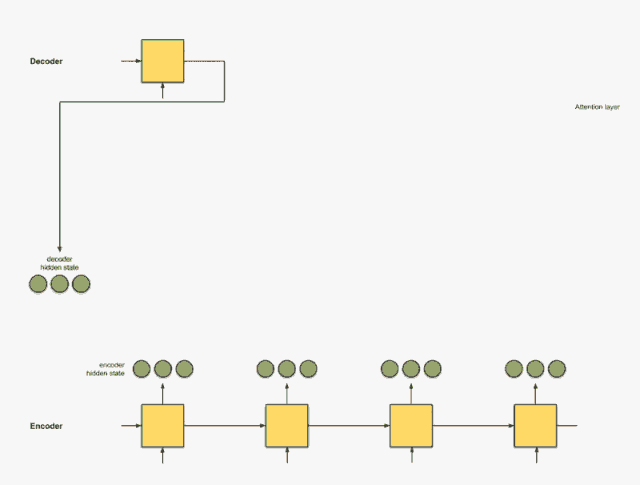decoder_hidden = [10, 5, 10]
encoder_hidden score
---------------------
[0, 1, 1]     15 (= 10×0 + 5×1 + 10×1, the dot product)
[5, 0, 1]     60
[1, 1, 0]     15
[0, 5, 1]     35

Step 2：將所有得分送入 softmax 層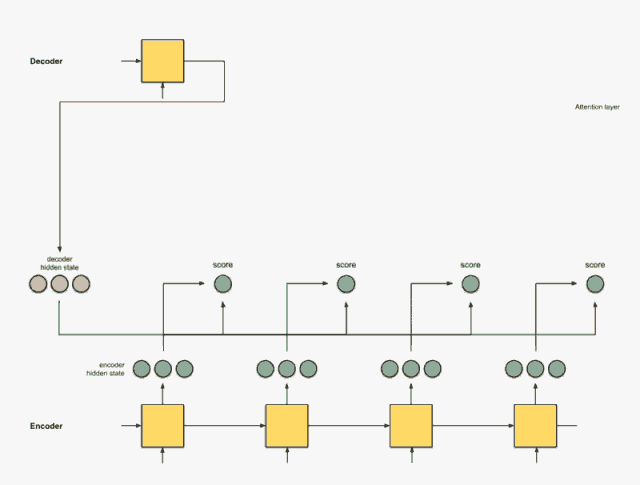encoder_hidden score score^
-----------------------------
[0, 1, 1]     15       0
[5, 0, 1]     60       1
[1, 1, 0]     15       0
[0, 5, 1]     35       0

Step 3：用每個 Encoder 的隱藏狀態乘以 softmax 之后的得分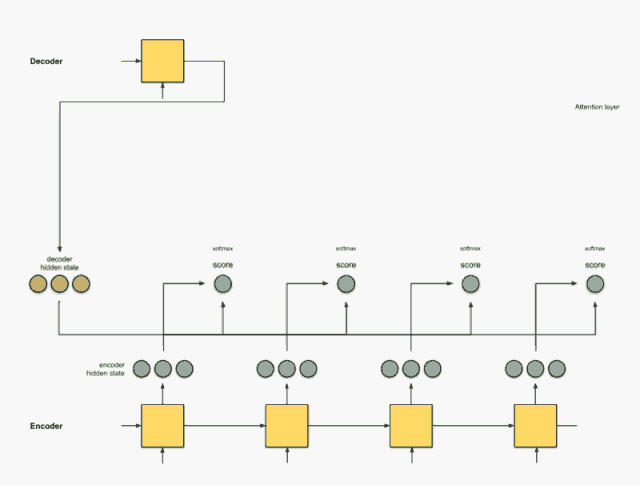encoder score score^ alignment
---------------------------------
[0, 1, 1]   15     0   [0, 0, 0]
[5, 0, 1]   60     1   [5, 0, 1]
[1, 1, 0]   15     0   [0, 0, 0]
[0, 5, 1]   35     0   [0, 0, 0]

Step 4：將所有對齊的向量進行累加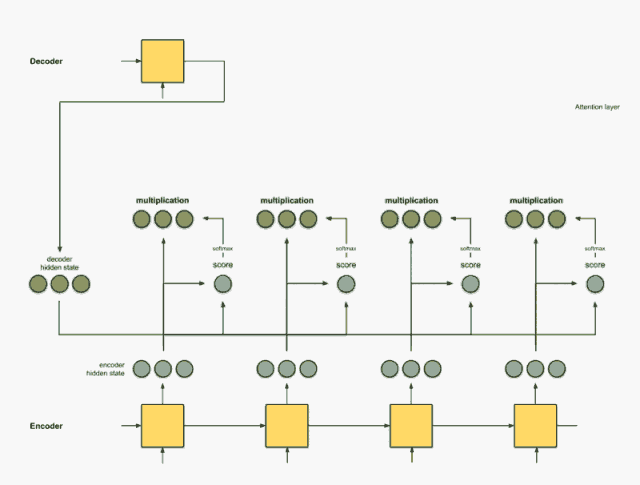Step 5：把上下文向量送到 Decoder 中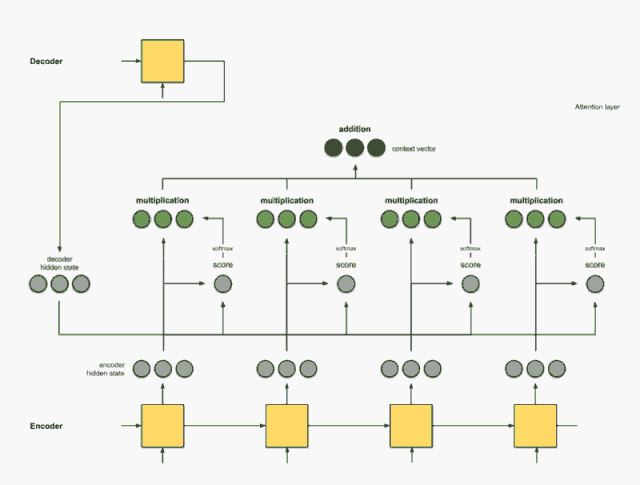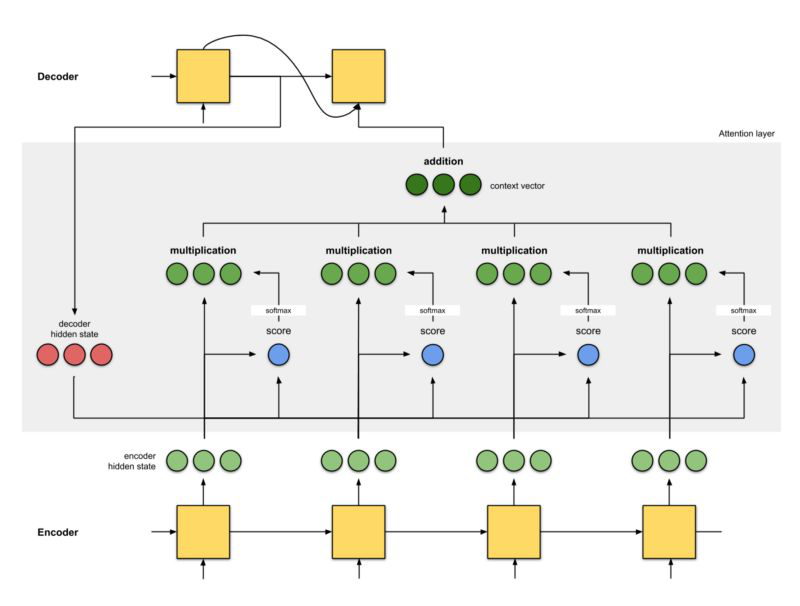# Attention 機制的優劣

• 一步到位的全局聯系捕捉，且關注了元素的局部聯系；attention 函數在計算 attention value 時，是進行序列的每一個元素和其它元素的對比，在這個過程中每一個元素間的距離都是一；而在時間序列 RNNs 中，元素的值是通過一步步遞推得到的長期依賴關系獲取的，而越長的序列捕捉長期依賴關系的能力就會越弱。

• 并行計算減少模型訓練時間；Attention 機制每一步的計算都不依賴于上一步的計算結果，因此可以并行處理。

• 模型復雜度小，參數少

# 參考資料

posted @ 2019-06-17 09:50  Maple17  閱讀(...)  評論(... 編輯 收藏